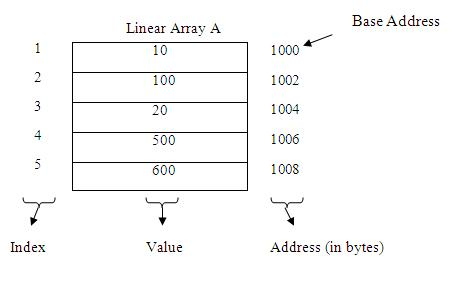The elements of linear array are stored in consecutive memory locations. The computer does not keep track of address of each element of array. It only keeps track of the base address of the array and on the basis of this base address the address or location of any element can be found. We can find out the location of any element by using following formula: `LOC (LA [K]) = Base (LA) + w (K – LB)` Here LOC (LA [K]) is the location of the Kth element of LA. Base (LA) is the base address of LA. w is the number of bytes taken by one element. K is the Kth element. LB is the lower bound.Suppose we want to find out Loc (A ). For it, we have: Base (A) = 1000 w = 2 bytes (Because an integer takes two bytes in the memory). K = 3 LB = 1 After putting these values in the given formula, we get: LOC (A ) = 1000 + 2 (3 – 1) = 1000 + 2 (2) = 1000 + 4 = 1004 Share this article   |    Print    |    Article read by 14799 times Author:Rohit kakria I am software developer Related Articles: No related article Related Interview Questions: No related interview question